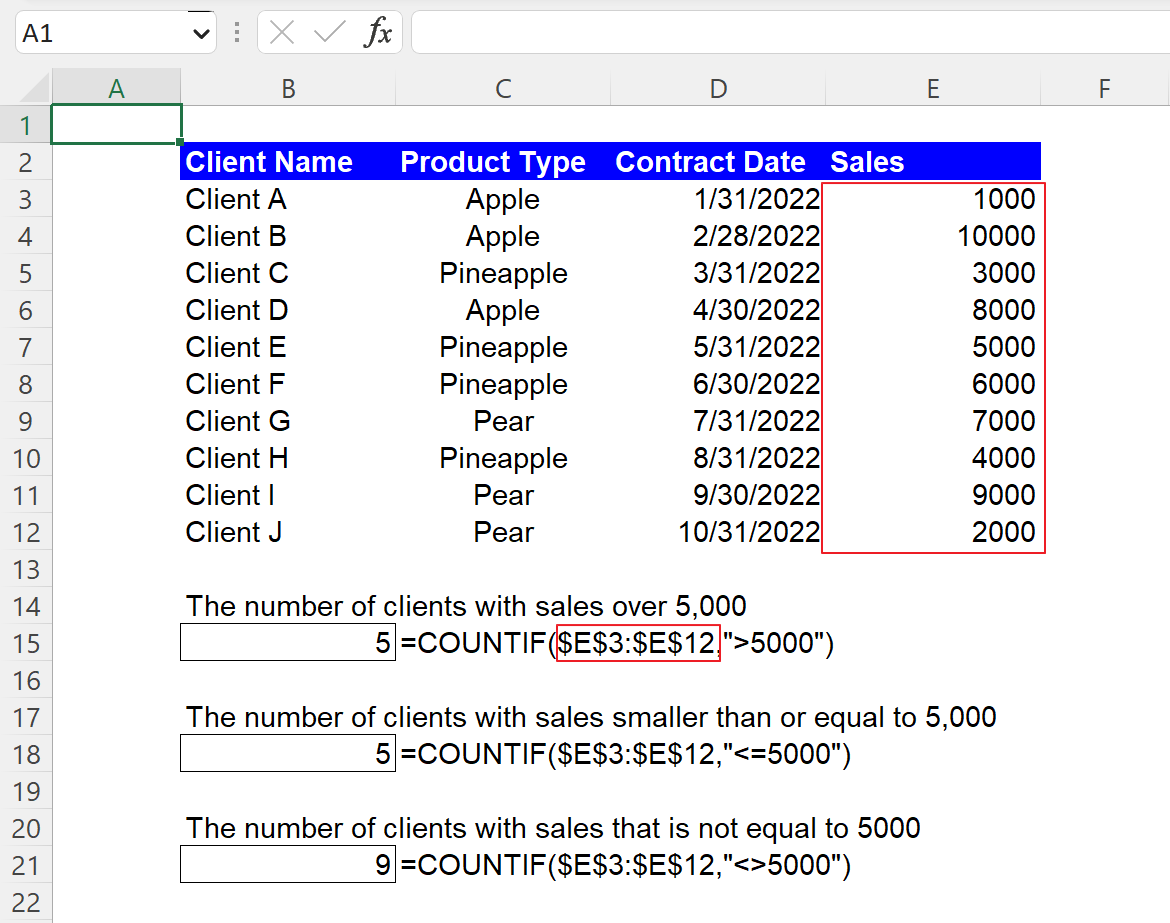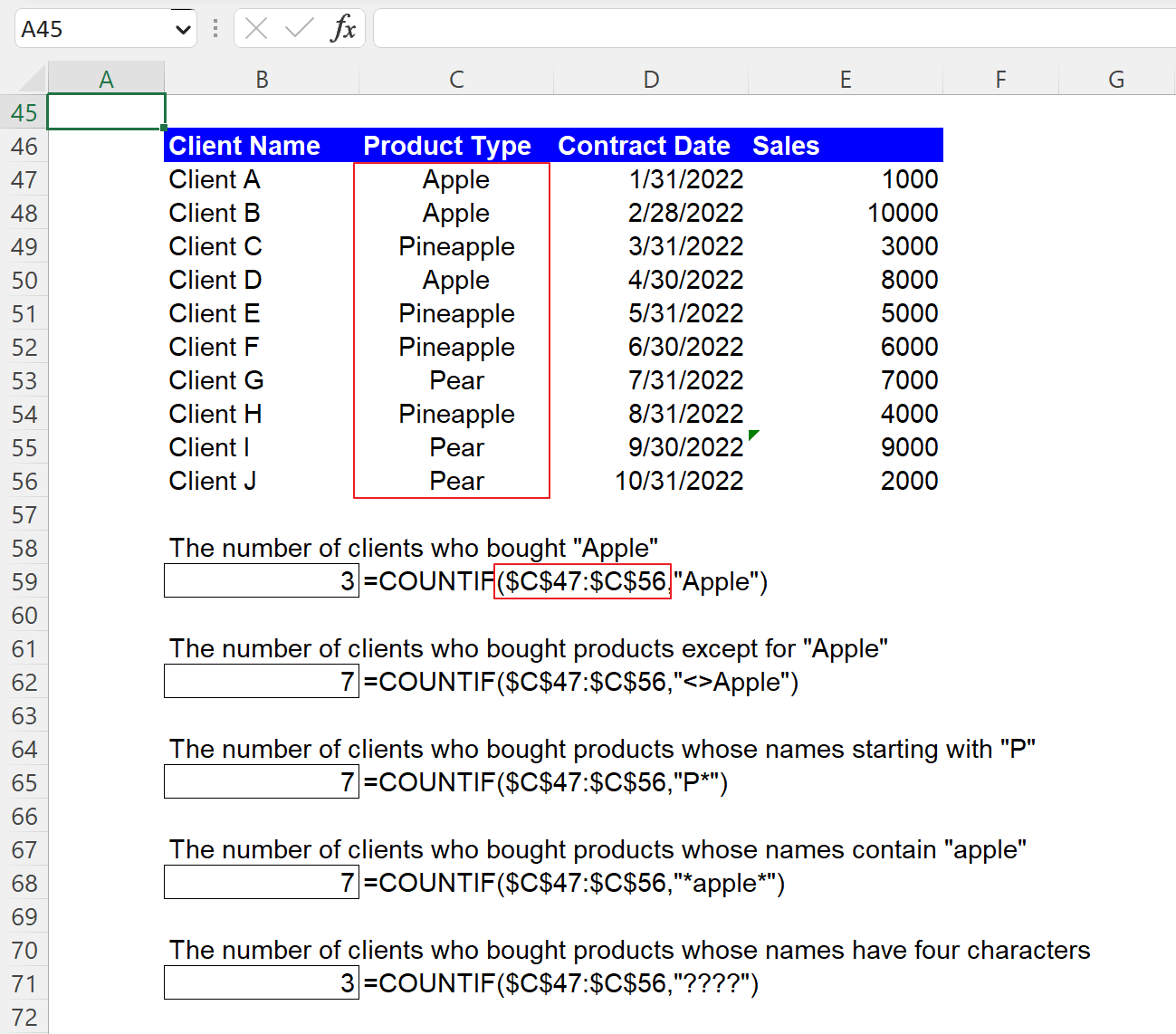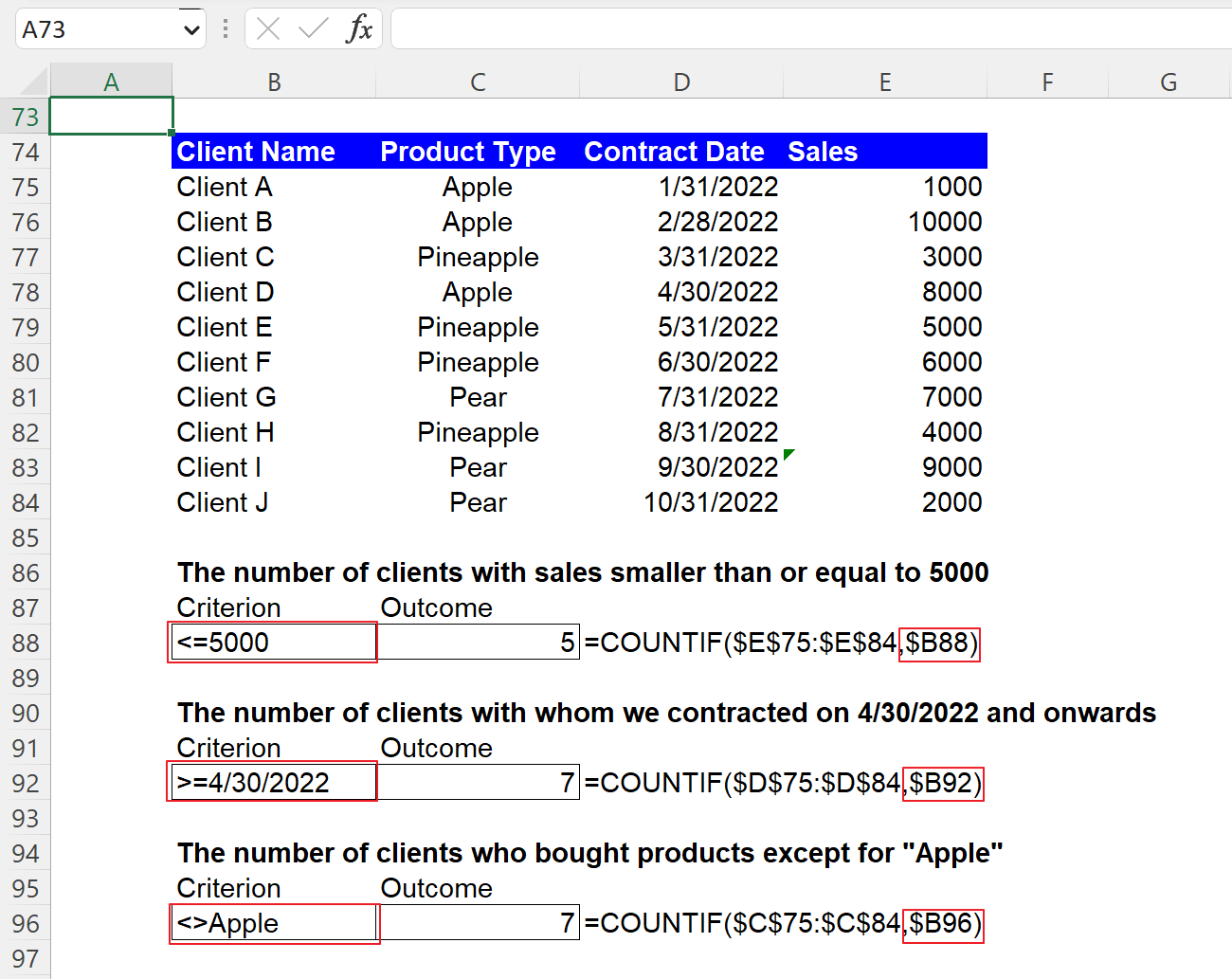Excel Formulas

# COUNTIF Function in Excel: Explained

In this article, you will learn how to use the COUNTIF formula in Excel.

## What is the COUNTIF function in Excel?

In Excel, the COUNTIF function is used to count the number of cells within a range that meet a specified criterion.

## When is the COUNTIF formula helpful in Excel?

The COUNTIF formula in Excel is helpful when you want to quickly count the number of cells in a range that meet a particular condition. For example, it can be used to count the number of items sold in a specific category, or the number of customers from a specific region. It's a powerful tool when working with large data sets, as it allows you to quickly and easily summarize data, identify trends, and make data-driven decisions.

## How to use the COUNTIF function in Excel

The syntax for the COUNTIF function is as follows:

``=COUNTIF(range, criteria)``

The "range" argument specifies the cells that you want to count, and the "criteria" argument is the condition that the cells must meet in order to be counted.

Note 1: Don’t forget to enclose a non-numeric value with double quotation marks when you include it as “criteria”.

Note 2: The COUNTIF formula is not case-sensitive. For instance, “apple” and “Apple” are considered the same by the formula when they are entered into the “criteria” argument.

Note 3: Zero value is returned when you expect a return larger than zero, ensure a correct “criteria” argument is incorporated in the formula. In that case, you should double-check whether the values in your dataset contain hidden characters, such as whitespace.

Note 4: When you try to find strings with greater than 255 characters, the COUNTIF formula may return an incorrect outcome.

## How to use the COUNTIF formula with a numeric condition

Before looking at sample COUNTIF formulas containing numeric criteria, get yourself familiarized with logic operators, such as “>N” (greater than N) and “<N” (less than N).

1. >N: Greater than N
2. >=N: Greater than or equal to N
3. <N: Less than N
4. <=N: Less than or equal to N
5. =N: Equal to N
6. <>N: Not equal to N

Assume you have the dataset in the picture below and want to compute the number of clients that meets a specific criterion, such as the number of clients with sales over 5,000. The formula and its arguments are as follows:

``=COUNTIF(\$E\$3:\$E\$12,”>5000”)``

"range": \$E\$3:\$E\$12

"criteria": ”>5000”

You can see other examples in the screenshot below as well.How to use the COUNTIF formula with a numeric criterion in Excel

Note that when you use an equal operator “=” with a specific number, you can directly input the particular number without the operator, as shown below. They return the same results.

``=COUNTIF(\$E\$3:\$E\$12,”=5000”)``
``=COUNTIF(\$E\$3:\$E\$12,5000)``

## How to use the COUNTIF function with a date criterion

You can use a date as the “criteria” argument in the COUNTIF function, almost similar to a number criterion. So, you can combine a logic operator with a date to create a condition as well. However, note that you need to enclose a date with quotation marks when you directly enter it in the formula, such as the following formula, which returns the number of clients with whom you contracted on 4/30/2022 and thereafter.

``=COUNTIF(\$D\$25:\$D\$34,”>=4/30/2022”)``

"range": \$D\$25:\$D\$34

"criteria": ”>=4/30/2022”

You can see two more sample formulas with date conditions in the following picture.

## How to use the COUNTIF formula with a text condition

You must remember that when you manually insert a text condition as the “criteria” argument, you always need to enclose them with double quotes, as you do for a date criterion. Also, you can use the operators, such as “<>” or the wildcards, such as “*” and “?” together with a text string to form a condition. For example, if you want to calculate the number of clients who bought “Apple”, you can apply the following formula to the dataset.

``=COUNTIF(\$C\$47:\$C\$56,”Apple”)``

"range": \$C\$47:\$C\$56

"criteria": ”Apple”

You should bear in mind that this formula is not case-sensitive. So, for example, you can get the same result when you use “apple” instead of “Apple” as “criteria” in the formula. The following picture contains four more sample SUMIF formulas with text conditions if you are interested.How to use the COUNTIF formula with a text criterion in Excel

## How to use the COUNTIF function with cell reference in Excel

Although you can manually enter “criteria”, as shown in the examples above, instead, you can use the cell reference to input the “criteria” argument. We recommend the cell reference approach because it gives you more visibility and clarity about the condition as the requirement can be seen in a cell and allows you to change the condition more quickly. You don’t need to put your cursor on the cell containing the formula or open the cell by hitting the F2 button to see in detail or revise the function. Note that you don’t need to enclose it with quotation marks when you put a criterion in a cell, as shown in the picture below.How to use the COUNTIF formula with cell reference in Excel

## What is the difference between the COUNTIF and COUNTIFS formulas in Excel?

The COUNTIF and COUNTIFS formulas in Excel count the number of cells in a range that meet a specific condition or criteria. However, the COUNTIF function counts cells that meet one condition in a range of cells, whereas the COUNTIFS function is used to count cells that meet multiple criteria across multiple ranges of cells. So, in summary, you should use the COUNTIF function when you have only one criterion and the COUNTIFS in case you have more than one criteria you want to apply.

## Analyze your live financial data in a snap in Google Sheets

Are you learning this formula to visualize financial data, build a financial model, or conduct financial analysis? In that case, LiveFlow may help you automate manual workflows, update numbers in real-time, and save time. You can access various financial templates on our website, from the simple Income Statement to Multi-Currency Consolidated Financial Statement. Are you interested in this product but are an Excel user? That’s not a problem at all. You can connect Google Sheets to Excel quickly.